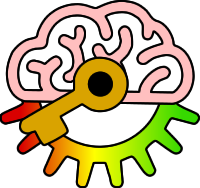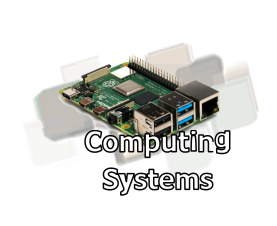# Y8

2i: Describe the NOT, AND, and OR logical operators.

Keywords:Test your self on these keywords and definitions using the games below or
KeywordDefinition
ANDLogic operator where the output is only True if both inputs are True
inputvalue that goes into a logic operator
Logic operatorWay of combining True/False values logically (AND, OR or NOT)
NOTLogic operator where the output is the opposite of the input
ORLogic operator where the output is True if either or both inputs are True
outputvalue that comes out as the result of a logic operator
Keyword games:
Resources: